# Pick theorem

Schwarz' lemma in invariant form

The following generalization of the Schwarz lemma. Let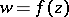be a bounded regular analytic function in the unit disc,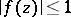for. Then for any points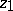and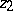inthe non-Euclidean distanceof their images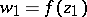and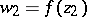does not exceed the non-Euclidean distance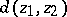, i.e.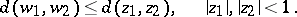(1)

One also has the inequality(2)

between the elements of non-Euclidean length (the differential form of Pick's theorem or the Schwarz lemma). Equality applies in (1) and (2) only ifis a Möbius function that mapsonto itself (cf. Fractional-linear mapping).

The non-Euclidean, or hyperbolic, distanceis the distance in Lobachevskii geometry betweenandwhenis the Lobachevskii plane and arcs of circles serve as Lobachevskii straight lines, these being orthogonal to the unit circle (Poincaré's model), and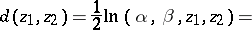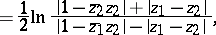whereis the cross ratio between the pointsandand the points of intersectionandof the Lobachevskii straight line passing throughandwith the unit circle (see Fig.).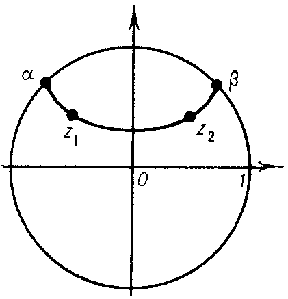Figure: p072700a

The non-Euclidean length of the image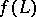of any rectifiable curveunder the mappingdoes not exceed the non-Euclidean length of.

The theorem was established by G. Pick ; a far-reaching generalization of it is provided by the principle of the hyperbolic metric (cf. Hyperbolic metric, principle of the). In geometric function theory these theorems provide bounds for various functionals related to mapping functions , .